# Test: Motion And Measurement Of Distances - 3

## 20 Questions MCQ Test Science Class 6 | Test: Motion And Measurement Of Distances - 3

Description
This mock test of Test: Motion And Measurement Of Distances - 3 for Class 6 helps you for every Class 6 entrance exam. This contains 20 Multiple Choice Questions for Class 6 Test: Motion And Measurement Of Distances - 3 (mcq) to study with solutions a complete question bank. The solved questions answers in this Test: Motion And Measurement Of Distances - 3 quiz give you a good mix of easy questions and tough questions. Class 6 students definitely take this Test: Motion And Measurement Of Distances - 3 exercise for a better result in the exam. You can find other Test: Motion And Measurement Of Distances - 3 extra questions, long questions & short questions for Class 6 on EduRev as well by searching above.
QUESTION: 1

### A cyclist moves from a certain point P and goes round a circle of radius a and reaches Q, exactly at the other side of the point P as shown in figure. The displacement of the cyclist would be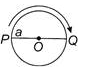Solution:

Displacement of the cyclist = Shortest distance between P and Q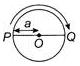= PO+OQ = a+a = 2a

QUESTION: 2

### Read the given statements and select the correct option, Statement 1: The displacement of a body may be zero, though distance can be finite. Statement 2: If a body moves such that finally it arrives at initial point, then displacement is zero while distance is finite.

Solution:

When a body moves from one position to another, the shortest distance measured between the initial and the final positions of the moving body in a particular direction is called displacement. The actual length of the path travelled by a moving body in a given interval of time is called the distance travelled by that body. So displacement of a body may be zero, though distance can be finite. For example, in case of circular motion if the body comes back to its initial position then distance travelled is finite but displacement is zero.

QUESTION: 3

### In circular motion, the

Solution:

When an object moves along a circular path, it is called circular motion. During such a motion, the direction of motion at any point is given by the tangent at that point which changes continuously.

QUESTION: 4

What causes a moving body to resist a change in its state of motion?

Solution:

The property of a body by virtue of which it resists any change in its state of rest or of motion in a straight line on its own is called its inertia.

QUESTION: 5

A rider on horse falls back when horse starts running all of a sudden because

Solution:

A rider on horse falls back when horse starts running, all of a sudden because the rider is in state of rest initially and later inertia of rest keeps the upper part of body at rest while lower part of the body moves forward with horse.

QUESTION: 6

One centimeter on a scale is divided into 20 equal divisions. The least count (minimum value) of this scale is

Solution:

As 20 divisions = 1 cm
∴ 1 division = 1/20 cm
= 0.05cm
= 0.5 mm
Hence, least count of this scale = 0.5 mm

QUESTION: 7

Match the following and select the correct option from the codes given below.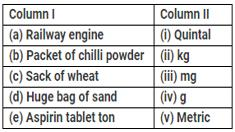Solution:
QUESTION: 8

Which of the following motions is/are periodic as well as oscillatory motion?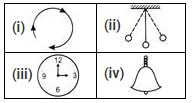Solution:

Only (ii) shows the periodic as well as oscillatory motion. It is showing to and fro as well as motion which is repeating after regular time interval.

QUESTION: 9

Which of the following statements is correct?

Solution:

Motion of soldiers on march past is rectilinear motion; Every oscillatory motion is also a periodic motion; Hockey player running after a ball is random motion.

QUESTION: 10

Four pieces of wooden sticks P, Q, R and S are placed along the length of 15 cm long scale as shown in figure. What is the average length of these sticks?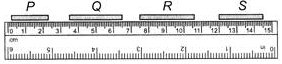Solution:

P = 2.1 cm; Q = 3.0 cm; R = 3.1 cm; S = 2.5 cm
Average length of these sticks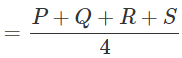= 2.6cm

QUESTION: 11

Direction: Observe the given situation and answer the following question.
Rahul and Ravi are playing in a ground. They start running from the same point X simultaneously in the ground and reach point Y at the same time by following paths marked 1 and 2 respectively, as shown in the figure.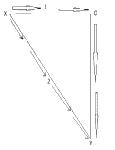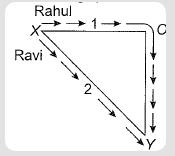Q. Which one of the following paths does show the displacement?

Solution:

Rahul takes path XOY and Ravi takes path XY. Displacement is minimum distance between initial and final point = XY.

QUESTION: 12

Rahul and Ravi are playing in a ground. They start running from the same point X simultaneously in the ground and reach point Y at the same time by following paths marked 1 and 2 respectively, as shown in the figure.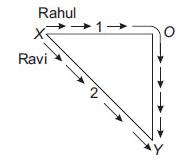Q. Which of the following is correct statement for the given situation?

Solution:

Rahul and Ravi both cover different distances XOY and XY respectively at the same time. Rahul covers more distance than Ravi in the same time. Hence Rahul covers longer distance with a higher speed.

QUESTION: 13

Which of the following statements is incorrect?

Solution:

Rectilinear motion is another name for straight-line motion.

QUESTION: 14

While measuring the length of a wooden box, the reading at one end is 1.5 cm and the other end is 4.7 cm. What is the length of the wooden box?

Solution:

The length of the wooden box = Difference between the reading at both the ends = 4.7−1.5 = 3.2 cm

QUESTION: 15

Motion of strings of a guitar shows
(i) oscillatory motion
(ii) vibratory motion
(iii) random motion
(iv) rotational motion

Solution:

Motion of strings of a guitar shows oscillatory as well as vibratory motion.

QUESTION: 16

Study the given Venn diagram, Which of the motions described by different bodies are most likely to be I, II and III?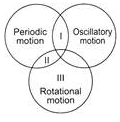Solution:

Option 1,2 and 3 are rotatory motion. Rotatory motion, also referred to as rotational motion or circular motion, is physical motion that happens when an object rotates or spins on an axis.

QUESTION: 17

Two identical metal balls A and 8 moving in opposite directions with different speeds hit each other at point X as shown in the figure. Changes will most likely appear in their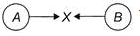1. Shapes
2. Speeds
3. Directions
4. Volumes

Solution:

In case of collision, changes are most likely to be appear in the speed and direction of both the balls.

QUESTION: 18

A pendulum swings backwards and forwards passing through Y, middle point of the oscillation. The first time the pendulum passes through Y, a stopwatch is started.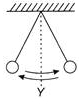The twenty-first time the pendulum pass through Y, the stopwatch is stopped. The reading is T. What is the time period of the pendulum?

Solution:

The time taken to complete one oscillation is called the time period of a pendulum.Here the time period is the time taken to move from Y to B then back to A and then to Y. The reading of stopwatch = T Number of oscillations = 10

∴  Time taken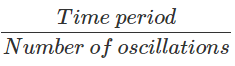= T/10

QUESTION: 19

Given figure shows a measuring cylinder (incm3) before and after the immersion of an irregular solid object. The volume of the object is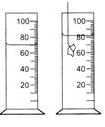Solution:

Initial volume of water in the cylinder, (before the immersion of the object)
V= 70cm3
Final volume in the cylinder (after the immersion of the object)
V= 82cm3
Hence, volume of the object immersed in the cylinder = Final volume - Initial volume
= 82 cm3−70 cm
= 12 cm3

QUESTION: 20

A piece of thread folded 6 times is placed along a 15 cm long measuring scale as shown in the figure. The length of the thread is between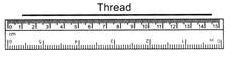Solution:

The length of the thread is between 0.50m-1.0m